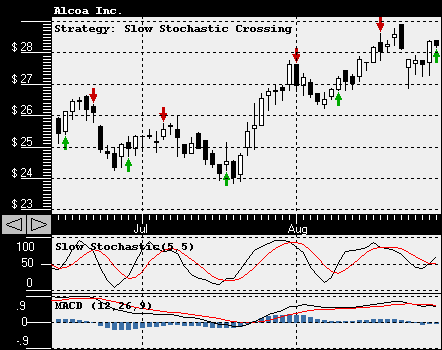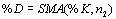# Slow Stochastic Oscillator

Slow Stochastic Oscillator is very similar to Fast Stochastic Oscillator. It is also a momentum indicator measuring the price of a security relative to the high/low range over a fixed period of time. The difference is that Slow Stochastic Oscillator averages lowest lows and highest highs of the stock on a fixed period of time in the process of calculation. This results in a smoother behavior of this indicator comparing to Fast Stochastic Oscillator.These are two typical ways to generate stock signals using Slow Stochastic Oscillator: buy when %K (black line) or %D (red line) is below 20 and sell when %K or %D is rises above 80 level; or buy when %K is crossing %D in an upward direction and sell when %K is crossing %D in a downward direction.

Slow Stochastic Oscillator is calculated using the following formula:here n1 and n2 are variable parameters and SMA is a simple moving average.